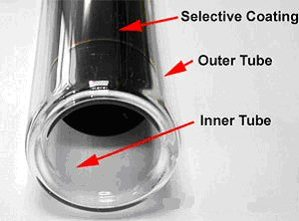# Thoughts on the Solar Energy Calculator

In my previous post, I outlined how to calculate the solar energy that reaches a solar panel in any orientation at any time. In the mean time, I created an emoncms input processor that calculates the momentary yield based on the increases of temperature in the water tank (I will describe that in a separate post). So comparing the calculated and actual yield, I was expecting to see  50-70% efficiency range, however I am seeing a yield that is higher than the calculated energy falling on the solar panel. I have verified that the actual yield readings are accurate by comparing with immersion heater and hand calculation so I focused on the solar energy calculator. I ran some checks and identified couple problems:

1. When I was setting the panel’s azimuth in the Calculator, I used phone and a compass application. Seems that it was quite off when I dobule checked, I then overlayed a compass on google satellite map and now fixed the correct panel azimuth
2. When calculating the surface of the panel, I used the following formula

Tube length*tube diameter*number of tubes

That however, isn’t entirely correct as the tubes are double and I need to calculate the inner tube diameter only, so I adjusted for that3. The tube length is 1.8m, but not all of it is exposed to the Sun, a small part of it is actually plugged in the solar tank, so that was my second adjustment

The last two changes resulted in a smaller panel surface calculation, and that was not helping in my case. So I researched further and an obvious fact that I have ommited became clear: The evacuated solar tubes act as a passive solar tracker. Due to the fact, that they are cylindrical in shape an they are placed with a significant gap on my collector, the solar energy reaches their surface at the optimal perpendicular angle for a much longer period compared to a flat panel. Here is a good explanation and some visual representation:Not only the angle is optimal, but there is a considerable amount of reflections being captured, resulting in  a considerably higher amount of energy falling on the tubes compared to a flat panel:So to conclude, my solar energy calculator is only good for flat surfaces and needs adjustment for solar tubes, given the above considerations. As a quick temporary fix, I have now assumed that the solar panel rotates along with the sun’s azimuth, but this is highly approximate. I will see what I can do to make this more elegant.